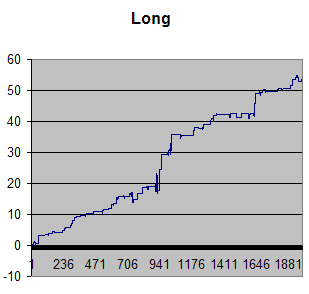# When XLP Diverges From Recent High And Low: A Close To Close Strategy

A while back I wrote about a daytrading strategy in SPY. This one works pretty well on XLP as well, with some modifications:

1. Calculate a 25 day average of the (High minus Low). That is the “ATR”.
2. Calculate the Low of the last 10 days.
3. Calculate the (C-L)/(H-L) ratio every day (IBS).
4. Calculate a band 2.5 times above the 10 day low using the average from point number 1 (ATR).
5. If XLP closes above the band in number 4, and point 3 has a higher value than 0.8, then go short at the close.
6. Exit on tomorrows close.

Vice versa for long except the value in number 3 must be below 0.33.

Test period from 2005 until 2013. Here is the results:

Long:

 Total % #Fills #Wins Average 53.57363 161 106 0.333

Profit curve:Short:

 Total P/L #Fills #Wins Average 49.03834 237 141 0.207

Equity curve:By the way, this strategy has been horrible in 2013 on the short side (live trading).